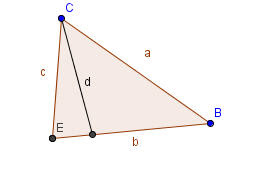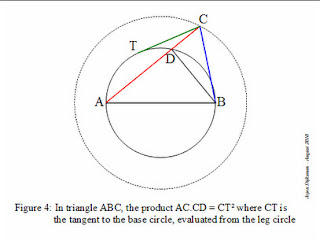## Monday 16 January 2023

### Almost Pythagorean, a collection on a theme

Years ago when I taught in Michigan I often gave talks on a collection of "Almost Pythagorean" relationships... things that reminded me of the classic. At one time I must have had a dozen or so, but I found a new one today on one of the blogs I follow. Arjen Dijksman is a Dutch Physicist whose blogs often tease out relations between physics and geometry,but his post is on the "Lost theorem about angular proportions" The property applies to any triangle in which one interior angle is twice another. Some beautiful extensions of chords in a unit circle produce a proof, but the part I liked was that if the sides and angles are labeled as shown :Then a2= c2+bc addendum, I noticed a couple of days after I posted this, that if you draw a segment call it d, cutting the angle formed by c and a so that c and d form the legs of an isosceles triangle, then the other triangle is also isosceles. One triangle with base angles of 2 theta, and the other with base angles of theta.Over time I continued to come across others, some from my reading and explorations, some from generous readers who shared.
Here are some of them as I first (mostly) wrote them.

Almost Pythagoras
For some reason I really like little simple geometric relations that remind me of the Pythagorean Theorem. I still remember the first time I saw 32 + 42=52 set next to 33+43+53=63and wondered.... Could it be????... Is it possible???? Oh go on, you know you are going to check... how could you resist? Just reminded of some other "almost Pythagorean" relations in geometry from an old (1932) Mathematics Teacher article...(Thank you, again, Dave Renfro) The Two Triangles formed when the median is drawn to any side are almost Pythagorean Treat the two sides not cut by the median as if they were the hypotenii (hypotenuses?) of a right triangle. Both are wrong, but in sum, they are right..That is, while neither of the following are true, AM2+MC2= AC2 AM2+MB2=AB2 Adding the two equations produces a true relation. AM2+MC2+AM2+MB2= AC2+AB2 The proof is pretty easy using the Law of Cosines. A second, and about as easy to prove.... Let G be the centroid of Triangle ABC, then AB2+BC2+CA2 = 3 (GA2+GB2+GC2) A third, which is, I believe, both necessary and sufficient to prove a parallelogram is that the sum of the squares of the diagonals is equal to the sum of the squares of the sides. And if it is not a parallelogram, but is a trapezoid, then the sum of the squares of the diagonals is equal to the sum of the squares of the non-parallel sides, plus twice the product of the parallel bases (sort of a law-of-cosines look-a-like). Recently saw the old Wizard of Oz mis-spoken statement of the Theorem by Ray Bolger as the Scarecrow posted at 360, so I thought this was a good time to include it for my students (and anyone else who has never seen it, or just wants to chuckle one more time). [
Play it for your students and see if they can catch all the mistakes...

and yet Another "Almost Pythagorean" Relation

I have written frequently about relationships that, for one reason or another, reminded me of the Pythagorean Theorem. (see here, and here, and here for example). After trying to write up all the ones I could think of, out of the blue I got a nice note from professor Robin Whitty who maintains the excellent "Theorem of the Day" web site. (If you are a high school teacher or student, it's a must see.) He had prepared a page on Des Cartes' Circle Theorem, and had included a link to my page on the subject (told you it was a good site). It was one more "almost Pythagorean" theorem. It's easiest to right if we consider the bend of a circle as the reciprocal of the radius, b= 1/r. With that notation adjustment, then for four circles with bends b1, b2,b3,b4, Des Cartes' Circle theorem says that one-half the square of the sum of the bends is equal to the sum of the squares of the bends.### Euler does an Almost Pythagorean Theorem

Today I found another while reading one of the wonderful articles by Ed Sandifer that were regularly featured in the MAA, "How Euler Did It."
This particular one was is titled "Beyond Isosceles Triangles".

This is about Euler's paper E324 -- Proprietates triangulorum, quorum anguli certam inter se tenent rationem (Properties of triangles for which certain angles have a ratio between themselves) which is not yet translated at The Euler Archive.

"We know lots about triangles for which Angle A = Angle B. Such triangles are isosceles, and we have known at least since Euclid that Angle A = Angle B exactly when a = b. In 1765, Euler studied a generalization of this situation. What happens if Angle B is some multiple of Angle A ?"
The one that caught my eye says "if the sides of a triangle satisfy the relation ac = bb- aa , then Angle B = 2 Angle A ." ..
I prefer it better as a2 + ac = b2.

Students should know a couple of candidates in which one angle is twice another.  The 30, 60. 90 for example, and an isosceles right triangle should both be candidates to confirm.

Euler goes on to prove that when Angle B is three times Angle A, then (b2 -a2)(b - a) =ac2 . Beautiful, but not quite so "Pythagorean"..

The geometry is clever, and probably clear enough for a really good high school geometry student to handle. Too many diagrams to copy here, so give it a read.
Sandifer says Euler continues through a ratio of 5 to 1, spots a pattern and extends the results to 13.

Sounds like a neat class project to me.

Another "Almost Pythagorean" RelationshipA while back Arjen Dijksman at "Physics Intuitions" posted a blog reflecting on my post about Almost Pythagorean Triangles . Then he went me one better with a new relation that is pretty incredible. In essence, he shows that for ANY triangle, ABC with opposite sides a, b, and c, there is a relation a2+b2=c2+2 t2, where t length of the tangent from point C to the circle with diameter AB. He has some nice clear graphics to show the development.. check it out..and with a change of sign the equation generalizes to triangles where c lies inside the circle with diameter AB. This is, of course, too close to the law of cosines to be ignored.. and it is easy to see that t2 = a*b*cos(C)... in fact as soon as we set t2=AC*DC, and recognize that angle BDC is a right angle, we have Cos(C)= DC/BC... thus AC*DC = AC*BC*(DC/BC)= ab Cos(C).

I know I have more out in the Wayback machine.  Maybe someday I'll find the energy to track them down.